Next: 3.3 Master Equation Method Up: 3 Simulation of Single Previous: 3.1.5 Method of Critical

# 3.2 Tunneling: a Stochastic Process

Quantum mechanics tells us the transmission probability and rate of one electron tunneling through a potential barrier, but has little to say about the statistics of many electrons tunneling. To make statements about the  many body problem a single-electron circuit represents, one has to include the formalism of statistical mechanics and stochastic processes. One can only speculate about the statistics governing tunneling. Nevertheless, the model discussed below gives very good experimental agreement, which confirms the model's soundness.

The first assumption is that electrons can not probe the past, that is they posses no memory, and thus their tunnel rate depends only on the momentary state of the system. This is exactly the criterion for a  Markov process . If one further assumes, that the system evolves at random times in a jump like fashion, as is the case with tunneling, one can describe such a system with a  ME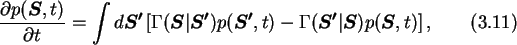where p(S,t) is the probability density function in state space, and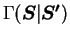denotes the transition rate from state S' to state S. If the states are discrete the ME becomes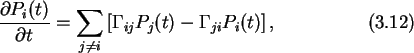where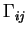denotes the transition rate from state j to state iand Pi(t) is the time dependent  occupation probability of state i. Fig. 3.5 is a typical state transition diagram for such a process.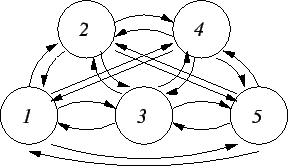The ME method for the simulation of SET circuits tries to solve (3.12) which is the general description of a SET circuit, numerically, as was done by L. Fonseca et al. . Taking the simplification one step further and considering only a single tunnel junction, where the number of tunneled electrons gives the state of the system, the state can only change to a neighboring state, since only one electron is assumed to tunnel at a time. Processes with this property are called  point or  birth-death processes  and can be visualized with a simple  state transition diagram Fig. 3.6.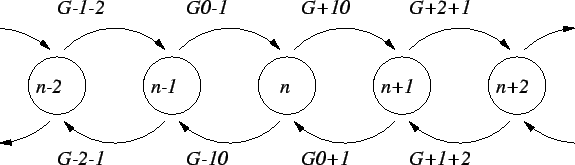Further assuming that states can only evolve in one direction, this is the case if only tunneling in one direction is considered, and that all states have an equal transition rate to the next state, which is the case when neglecting any charging effects, brings one to the so called  Poisson process, Fig. 3.7.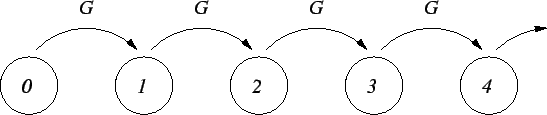The Poisson process starts at n=0 and evolves monotonously to higher state numbers. The transition rate is the same for every state. The ME for the Poisson process is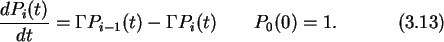This first order differential equation with constant coefficients may be solved by Laplace transforming both sides.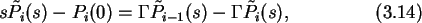where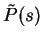is the Laplace transform of P(t). Using the initial conditions P0(0)=1 and Pi>0(0)=0 gives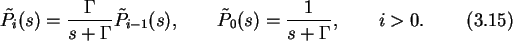This set of recursive equations for the state probability Laplace transform is easily solved by induction.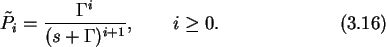Taking the inverse Laplace transform, one finds that forand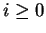,This is the well known  Poisson distribution. Building on the stochastic description of a single tunnel junction with the Poisson process, it is possible to use a MC method for the simulation of SET circuits.Next: 3.3 Master Equation Method Up: 3 Simulation of Single Previous: 3.1.5 Method of Critical

Christoph Wasshuber